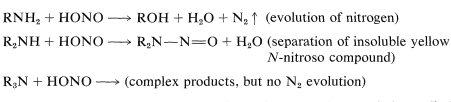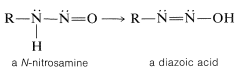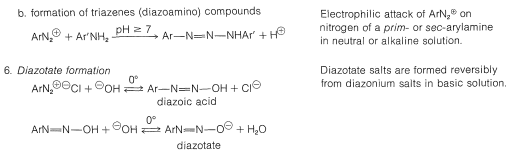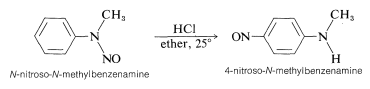# 23.10: Amines with Nitrous Acid

$$\newcommand{\vecs}{\overset { \rightharpoonup} {\mathbf{#1}} }$$ $$\newcommand{\vecd}{\overset{-\!-\!\rightharpoonup}{\vphantom{a}\smash {#1}}}$$$$\newcommand{\id}{\mathrm{id}}$$ $$\newcommand{\Span}{\mathrm{span}}$$ $$\newcommand{\kernel}{\mathrm{null}\,}$$ $$\newcommand{\range}{\mathrm{range}\,}$$ $$\newcommand{\RealPart}{\mathrm{Re}}$$ $$\newcommand{\ImaginaryPart}{\mathrm{Im}}$$ $$\newcommand{\Argument}{\mathrm{Arg}}$$ $$\newcommand{\norm}{\| #1 \|}$$ $$\newcommand{\inner}{\langle #1, #2 \rangle}$$ $$\newcommand{\Span}{\mathrm{span}}$$ $$\newcommand{\id}{\mathrm{id}}$$ $$\newcommand{\Span}{\mathrm{span}}$$ $$\newcommand{\kernel}{\mathrm{null}\,}$$ $$\newcommand{\range}{\mathrm{range}\,}$$ $$\newcommand{\RealPart}{\mathrm{Re}}$$ $$\newcommand{\ImaginaryPart}{\mathrm{Im}}$$ $$\newcommand{\Argument}{\mathrm{Arg}}$$ $$\newcommand{\norm}{\| #1 \|}$$ $$\newcommand{\inner}{\langle #1, #2 \rangle}$$ $$\newcommand{\Span}{\mathrm{span}}$$

## Alkanamines with Nitrous Acid

Some of the most important reactions of amines are brought about by nitrous acid $$\left( \ce{HONO} \right)$$. The character of the products depends very much on whether the amine is primary, secondary, or tertiary. In fact, nitrous acid is a useful reagent to determine whether a particular amine is primary, secondary, or tertiary. With primary amines nitrous acid results in evolution of nitrogen gas; with secondary amines insoluble yellow liquids or solid $$\ce{N}$$-nitroso compounds, $$\ce{R_2N-N=O}$$, separate; tertiary alkanamines dissolve in and react with nitrous acid solutions without evolution of nitrogen, usually to give complex products:Nitrous acid is unstable and always is prepared as needed, usually by mixing a solution of sodium nitrite, $$\ce{NaNO_2}$$, with a strong acid at $$0^\text{o}$$. These conditions provide a source of $$^\oplus \ce{NO}$$, which is transferred readily to the nucleophilic nitrogen of the amine:With this common key step, why do amines react differently with nitrous acid depending on their degree of substitution? The answer can bee seen from the reactions that are most easily possible for the $$\ce{-} \overset{\oplus}{\ce{N}} \ce{-NO}$$ intermediate. Clearly, if there is a hydrogen on the positive nitrogen, it can be lost as a proton and a $$\ce{N}$$-nitrosamine formed:With a secondary amine, the reaction stops here, with formation of $$\ce{R_2N-NO}$$, and because these substances are very weak bases, they are insoluble in dilute aqueous acids. They are characteristically yellow or orange-yellow solids or oils.

A tertiary amine$$\cdot \overset{\oplus}{\ce{N}} \ce{O}$$ complex, $$\ce{R_3} \overset{\oplus}{\ce{N}} \ce{-NO}$$, cannot lose a proton from nitrogen, but instead may lose a proton from carbon and go on to form complex products.

With a primary amine, the initially formed $$\ce{N}$$-nitrosamine can undergo a proton shift by a sequence analogous to interconversion of a ketone to an enol. The product is called a diazoic acid:Some diazoic acids form salts that are quite stable, but the acids themselves usually decompose rapidly to diazonium ions:Diazonium salts can be regarded as combinations of carbocations $$\ce{R}^\oplus$$ with $$\ce{N_2}$$ and, because of the considerable stability of nitrogen in the form of $$\ce{N_2}$$, we would expect diazonium salts to decompose readily with evolution of nitrogen and formation of carbocations. This expectation is realized, and diazonium salts normally decompose in this manner in water solution. The aliphatic diazonium ions decompose so rapidly that their presence can only be inferred from the fact that the products are typically those of reactions of carbocations:With propanamine, loss of nitrogen from the diazonium ion gives the very poorly stabilized propyl cation, which then undergoes a variety of reactions that are consistent with the carbocation reactions discussed previously (see Sections 8-9B and 15-5E):The isopropyl cation formed by rearrangement undergoes substitution and elimination like the propyl cation. About half of the products arise from isopropyl cations.

There is one exceptional reaction of the propyl cation that involves 1,3-elimination and formation of about $$10\%$$ of cyclopropane:Clearly, the plethora of products to be expected, particularly those resulting from rearrangement, prevents the reaction of the simple primary amines with nitrous acid from having any substantial synthetic utility.

## Arenamines with Nitrous Acid. Arenediazonium Salts

Unlike primary alkylamines, primary arenamines react with nitrous acid at $$0^\text{o}$$ to give diazonium salts that, in most cases, are stable enough to be isolated as crystalline $$\ce{BF_4^-}$$ salts. Other salts can be isolated, but some of these, such as benzenediazonium chloride, in the solid state may decompose with explosive violence.The reason for the greater stability of arenediazonium salts compared with alkanediazonium salts appears to be related to the difficulty of forming aryl carbocations (Section 14-6A). Even the gain in energy associated with having nitrogen as the leaving group is not sufficient to make aryl cations form readily, although solvolysis of arenediazonium ions in water does proceed by an $$S_\text{N}1$$ mechanism:This reaction has general utility for replacement of aromatic amino groups by hydroxyl groups. In contrast to the behavior of alkylamines, no rearrangements occur.

Generally, diazonium salts from arenamines are much more useful intermediates than diazonium salts from alkanamines. In fact, arenediazonium salts provide the only substances that undergo nucleophilic substitution reactions on the aromatic ring under mild conditions, without the necessity of having activating groups, such as nitro or cyano, in the ortho or para position. The most important reactions of this type include the replacement of the diazonium group by nucleophiles such as $$\ce{Cl}^\ominus$$, $$\ce{Br}^\ominus$$, $$\ce{I}^\ominus$$, $$\ce{CN}^\ominus$$, $$\ce{NO_2^-}$$, and these reactions lead to the formation of aryl halogen, cyano, and nitro compounds. Most of these reactions require cuprous ions, $$\ce{Cu}$$(I), as catalysts. The method is known as the Sandmeyer reaction. The following examples illustrate how a primary arenamine can be converted to a variety of different groups by way of its diazonium salt:Aryl fluorides also may be prepared from arenamines by way of diazonium salts if the procedure is slightly modified. The amine is diazotized with nitrous acid in the usual way; then fluoroboric acid or a fluoroborate salt is added, which usually causes precipitation of a sparingly soluble diazonium fluoroborate. The salt is collected and thoroughly dried, then carefully heated to the decomposition point - the products being an aryl fluoride, nitrogen, and boron trifluoride:

$\ce{C_6H_5} \overset{\oplus}{\ce{N}} \: \overset{\ominus}{\ce{BF_4}} \overset{\text{heat}}{\longrightarrow} \ce{C_6H_5F} + \ce{N_2} + \ce{BF_3}$

This reaction is known as the Schiemann reaction. An example (which gives a better than usual yield) follows:Later in the chapter we shall see that amines can be prepared by the reduction of nitro compounds, which permits the following sequence of reactions:

$\ce{ArH} \overset{\ce{HNO_3}}{\longrightarrow} \ce{ArNO_2} \overset{\left[ \ce{H} \right]}{\longrightarrow} \ce{ArNH_2} \overset{\ce{HONO}}{\longrightarrow} \ce{ArN_2^+} \underset{-\ce{N_2}}{\overset{\ce{CuX}}{\longrightarrow}} \ce{ArX}$

This sequence is especially useful to introduce groups or produce orientations of substituents that may not be possible by direct substitution.

The Sandmeyer group of reactions is an example of the production of nucleophilic substitution by way of radical intermediates (see Section 14-10A):This mechanism is supported by the fact that $$\ce{Cu}$$(II) is important in the formation of $$\ce{C_6H_5X}$$. If the concentration of $$\ce{Cu}$$(II) is kept very low so as to slow down conversion of $$\ce{C_6H_5} \cdot$$ to $$\ce{C_6H_5^+}$$, and a compound with a reactive double bond is present, then products are formed by attack of $$\ce{C_6H_5} \cdot$$ on the double bond. This is called the Meerwein reaction:

$\ce{C_6H_5} \overset{\oplus}{\ce{N_2}} \: \overset{\ominus}{\ce{X}} + \ce{CH_2=CHCN} \overset{\ce{Cu} \left( \text{I} \right)}{\longrightarrow} \ce{C_6H_5CH_2-CHXCN} + \ce{N_2}$

Iodide ion appears to be a good enough reducing agent to form $$\ce{C_6H_5} \cdot$$ without the intervention of $$\ce{Cu}$$(I); considerable $$\ce{I_2}$$ usually is formed in the reaction:

\begin{align} \ce{C_6H_5} \overset{\oplus}{\ce{N_2}} + \overset{\ominus}{\ce{I}} &\rightarrow \ce{C_6H_5} \cdot + \ce{N_2} + \ce{I} \cdot \\ \ce{C_6H_5} \cdot + \ce{I} \cdot &\rightarrow \ce{C_6H_5I} \\ 2 \ce{I} \cdot &\rightarrow \ce{I_2} \end{align}

Secondary arenamines react with nitrous acid to form $$\ce{N}$$-nitroso compounds while tertiary arenamines undergo electrophilic substitution with $$\ce{NO}^\oplus$$ if they have an unsubstituted para position:## Diazo Coupling Reactions

Not all reactions of diazonium ions involve cleavage of the $$\ce{C-N}$$ bond. An important group of reactions of arenediazonium ions involves aromatic substitution by the diazonium ion acting as an electrophilic agent to yield azo compounds, $$\ce{Ar-N=N-Ar}$$:This reaction is highly sensitive to the nature of the substituent $$\ce{X}$$, and coupling to benzene derivatives normally occurs only when $$\ce{X}$$ is a strongly electron-donating group such as $$\ce{-O}^\ominus$$, $$\ce{-N(CH_3)_2}$$, and $$\ce{-OH}$$. However, coupling with $$\ce{X} = \ce{-OCH_3}$$ may take place with particularly active diazonium ions.

Diazo coupling has considerable technical value, because the azo compounds that are produced are highly colored. Many are used as fabric dyes and for other coloring purposes. A typical example of diazo coupling is formation of 4-$$\ce{N}$$,$$\ce{N}$$-dimethylaminoazobenzene from benzenediazonium chloride and $$\ce{N}$$,$$\ce{N}$$-dimethylbenzenamine:The product once was used to color edible fats and therefore was known as "Butter Yellow", but its use to color food is prohibited because it is reported to be a potent liver carcinogen for rats.

The pH used for diazo coupling of amines is very important in determining the nature of the products. Under near-neutral conditions the diazonium ion may attack the nitrogen of the arenamine rather than a ring carbon. In this event a diazoamino compound, a triazene, $$\ce{-N=N-N}-$$, is formed:The reaction is readily reversed if the pH is lowered sufficiently.

As you see from this brief discussion of arenediazonium salts, their chemistry is complex. It is inappropriate to discuss all of their many reactions here, but a summary of the most important types of reactions is given in Table 23-4.

Table 23-4: Summary of Reactions of Arenediazonium Salts## Rearrangements of $$\ce{N}$$-Substituted Arenamines

A secondary arenamine behaves like a secondary alkanamine in reacting with nitrous acid to give an $$\ce{N}$$-nitrosamine. However, when treated with an acid the $$\ce{N}$$-nitrosamine rearranges:This is one example of a group of formally related rearrangements in which a substituent, $$\ce{Y}$$, attached to the nitrogen of a benzenamine derivative migrates to the ortho or para positions of the aromatic ring under the influence of acid:Rearrangement occurs most readily when $$\ce{Y}$$ is a strongly electron-attracting group and the $$\ce{N-Y}$$ bond that is broken is not as strong as the $$\ce{C-Y}$$ bond that is formed. A few of the many examples of this type of reaction follow: